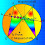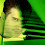## Sunday, September 8, 2013

### Geometry Problem 920: Triangle, Angles, 10, 20, 30 Degrees, Congruence

Level: Mathematics Education, High School, Honors Geometry, College.

This entry contributed by Erina-NJ.
Click the figure below to see the complete problem 920.1.I think that Ceva's trigonometric theorem gives:sin(30)/sin(10)*sin(x)/sin(120-x)=1 which yields, x=20° and /_BCD=100° as may ascertained from the diagram above.
In any case, what we are looking for is a synthetic solution, aren't we?

2.(Thank you once again Antony Gutierrez)
Problem 920. Pl refer to my earlier post:
By Ceva,
sin DAB. sin DBC. sin DCA = sin DAC. sin DBA. sin DCA
sin 10°.sin 30°.sin x = sin 10°.sin 10°.sin(120°-x).(This line is corrected)
So sin 30°.sin x = sin 10°.sin(120° - x).
Since sin 30° = 1/2 and sin(120°-x)= Sin(90°+30°-x)= cos(30°-x)
sin x = 2 sin 10°.cos(30°-x)
(OR)
By sine rule:
sin x / sin 10° = AD/DC = DB/DC = sin(120°-x)/sin 30° = 2 sin(120°-x)
So sin x = 2 sin 10°.sin(120°-x)= 2 sin 10°.cos(30°-x)
By inspection x = 20° satisfies the above equation.
P.S.:Is there a simple way of solving the above trig equation?

1.Problem 20 From Erina Nj To Pravin

Sinx = 2 sin10 cos (30-x)
sinx = sin (40-x)- sin (20-x)
sin (40-x)- sinx= Sin (20-x)
2sin (20-x) cos 20-sin (20-x) =0
sin(20-x) [2cos20-1] =0
2cos20-1 different from 0 => sin(20-x) = 0
X=20

2.@Anonymous, how about a synthetic solution to this exvellent problem?

3.http://www.mathematica.gr/forum/viewtopic.php?f=22&t=40350

4.can you solve using elemtery geomitry

1.MANOLOUDIS APOSTOLIS
4th HIGH SCHOOL OF KORYDALLOS -PIRAEUS-GREECE
PROBLEM 920
SOLUTION 1

E is a point of AC. Then <EBC=10 degrees so <DBE=20 degrees .If O the pericenter of triangle ABE then OA=OE=OB=AE because <AOE=2×<ABE=2×30=60 degrees and <OAB=<OBA= 40 degrees. Also DA=DB so OD is perpendicular to AB and OD is vertical to the middle of AB hence <AOD=50 degrees= <OAD thus DO=DA,OE=AE so tri ADE=tri ODE.
So <DEA = 60/2= 30 degrees = <DBC so DECB is inscribable hence <DCA=<DCE=<DBA= 20 degrees

SECOND SOLUTION
Let E, F the symmetrical of point A to DB and BC respectively. Then DA=DE=DB, AB=BE=BF, <CBF=<CBA= 40 degrees, <CFB= <CAB= 20 degrees. Then B is the pericenter of triangle AEF so <AFE =10 degrees so triangle EBF is equilateral. So DE=DB, EF=BF so DF is vertical to the middle of EB. We conclude that <DFA= 20 degrees, <DFC= 10 degrees= <DAC
so ADCF is inscribable hence <DCA=<DFA= 20 degrees

5.It seems the following proof it's not among the given ones: Take E the reflection of C in AD; easily BE=CE. Construct the equilateral triangle CED' inside the Langley triangle ACE; D'E=BE and <ABD'=10 degs, hence D'=D. Now <ACD=<AED=20.

Happy New Year!

6.Excellent work Apostolis and Stan in finding these pure geometry proofs

7.Thank you very much for your nice words Sumith